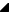﻿ ParseRotateMatrix(String) Method# ParseRotateMatrix(String) Method

Parses the input string for a rotation matrix definition ( rotate-angle [cx cy]), which specifies a rotation by degrees about a given point.

If optional parameters cx and cy are not supplied, the rotate is about the origin of the current user coordinate system. The operation corresponds to the matrix [cos(a) sin(a) -sin(a) cos( a) 0 0].

If optional parameters cx and cy are supplied, the rotate is about the point (cx, cy). The operation represents the equivalent of the following specification: translate(cx, cy)Syntax
 Delphi function ParseRotateMatrix( S: String ): TRSMatrix;

Parameters

S

Type: String

#### Return Value

Type: TRSMatrixSee Also